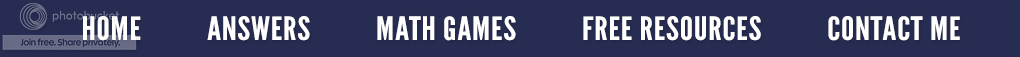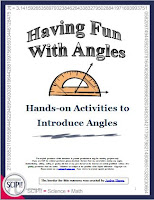### It Depends on the Angle - Complimentary and Supplementary Angles

My Basic Algebra Concepts class just started a brief chapter on geometry...my favorite to teach! We are currently working on angles, and as we went through the definitions, I noticed my students were having difficulty distinguishing complimentary from supplementary angles. Since most of my students are visual learners, I had to come up with something that would jog their memory.

The definition states that complementary angles are any two angles whose sum is 90°. (The angles do not have to be next to each other to be complementary.) As seen in the diagram on the left, a 30° angle + a 60° angle = 90° so they are complementary angles. Notice that the two angles form a right angle or 1/4 of a circle.

If I write the word complementary and change the first letter "C" into the number nine and I think of the letter "O" as the number zero, I have a memory trick my mathematical brain can remember.Supplementary Angles are two angles whose sum is 180°. Again, the two angles do not have to be together to be supplementary, just so long as the total is 180 degrees. In the illustration on your right, a 110° angle + a 70° angle = 180°; so, they are supplementary angles. Together, they form a straight angle or 1/2 of a circle.

If I write the word supplementary and alter the "S" so it looks like an 8, I can mentally imagine 180°.

Since there are so many puns for geometric terms.﻿ I have to share a bit of geometry humor. (My students endure many geometry jokes!)Only \$3.25
You might be interested in a variety of hands-on ideas on how to introduce angles to your students. Check out this resource.  It explains how to construct different kinds of angles (acute, obtuse, right, straight) using items such as coffee filters, plastic plates, and your fingers. Each item or manipulative is inexpensive, easy to make, and simple for students to use. All of the activities are hands-on and work well for kinesthetic, logical, spatial, and/or visual learners.
﻿